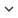ENGCongress
Congress 1080
Author/s
• Adrián Arias, Eduardo Sánchez and Luis Martínez
Source
• European Retina Meeting. Brighton, UK. 2015
Research Areas

# Effects of retinal DoG filters on natural images in terms of contrast, entropy and spatial correlation

This paper aims at analyzing the effects of retinal discrete Difference-of-Gaussians (DoG) filters on natural images. The filters were generated by means of a discretization process applied to the continuous DoG models obtained empirically from Retinal Ganglion Cells (RGCs) by Enroth-Cugell and Robson (1966). The results indicate that the sampling of a continuous DoG function, which models the input-output relationship of a RGC, determines the behavior of the corresponding discrete DoG kernel. The discretization process could yield filters with either a band pass or a high-frequency enhancing behavior. In order to analyze the differences among the operations carried out by such different filters we have resorted to the Gray-Level Co-occurrence Matrix (GLCM) and three image descriptors: contrast, entropy and spatial correlation. First, we derived a set of discrete DoG kernels from each continuous DoG function; then, we calculated the difference between the input and output values of each descriptor on each of those kernels.Our findings indicate that (1) each discrete kernel modifies the input contrast, entropy and spatial correlation in a different way; (2) the differences of entropy and contrast are logarithmically related; (3) the differences of contrast and spatial correlation are, on the other hand, linearly related with a negative slope; (4) contrast-enhancing kernels tend to reduce the spatial correlation and increase the entropy of the output; and (5) the output of contrast-enhancing kernels tends to increase both the spatial correlation and the entropy of low-contrast inputs. The relationships between contrast, entropy and spatial correlation were further tested on a natural image dataset, containing both natural scene and urban examples. The results showed similar dependencies between these descriptors. This suggests that the different kernels that a RGC could generate by pulling a different set of inputs preserve the statistics of the visual input. However, enhancing average contrast usually comes at the expense of increasing entropy and reducing spatial correlations. Thus, images with higher entropies would require longer size codes; i.e the amount of code (spikes) needed to represent the image would be increased rather than reduced. Moreover, in terms of machine learning, removing spatial correlations could hinder the process of learning visual patterns. Therefore, the optimal discretization of the DoG models would have to satisfy a trade-off between contrast, entropy and spatial correlation.